Win up to 100% scholarship on Aakash BYJU'S JEE/NEET courses with ABNAT Win up to 100% scholarship on Aakash BYJU'S JEE/NEET courses with ABNAT

# Straight Lines

A straight line is a line that is not curved or bent. All the basic and advanced concepts related to straight lines are covered here on this page. This lesson can also be downloaded as a PDF which helps students to refer to the concepts in offline mode.

## What Is a Straight Line?

A line is a geometry object characterised under zero width object that extends on both sides. A straight line is just a line with no curves. So, a line that extends to both sides to infinity and has no curves is called a straight line.

## Equation of a Straight Line

The general equation of a straight line is given below:

ax + by + c = 0

Where x and y are variables, a, b, and c are constants.

Slope:-

The equation of a straight line in slope-intercept form is given by:

y = mx + c

Here, m denotes the slope of the line, and c is the y-intercept.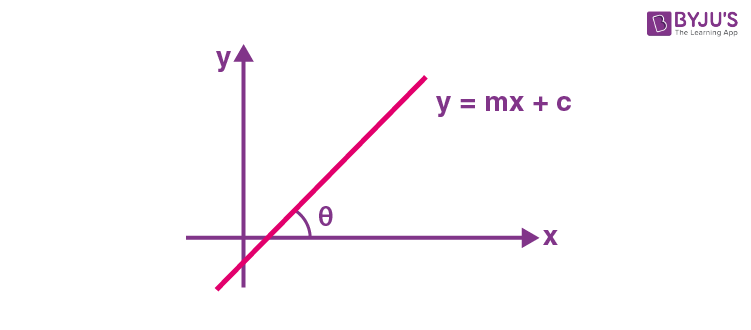When the angle with + ve x-axis ‘tan θ’ is called the slope of a straight line.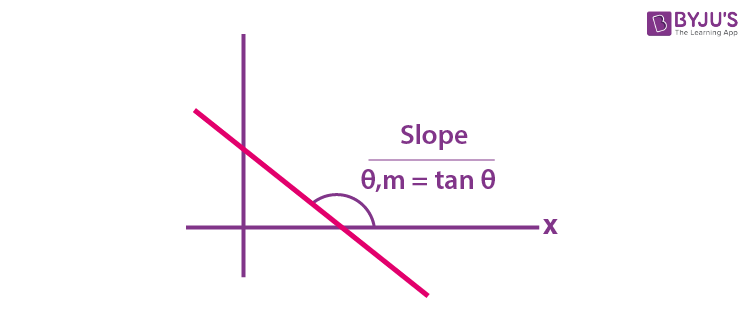Note 1 – If the line is horizontal, then slope = 0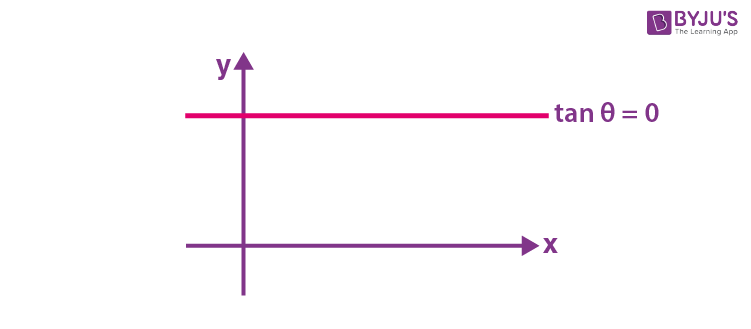Note 2 – If the line is perpendicular to the x-axis, i.e., vertical, then the slope is undefined.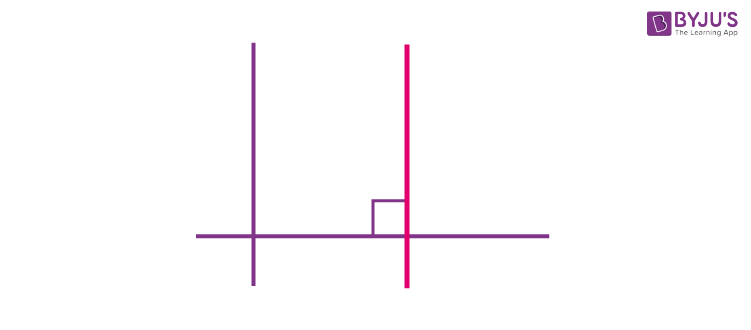Slope = 1/0 = = tan ⁡π/2

Note 3 – If the line is passing through any of two points, then the slope is

$$\begin{array}{l}tan\ \theta=\frac{y_2-y_1}{x_2-x_1}\end{array}$$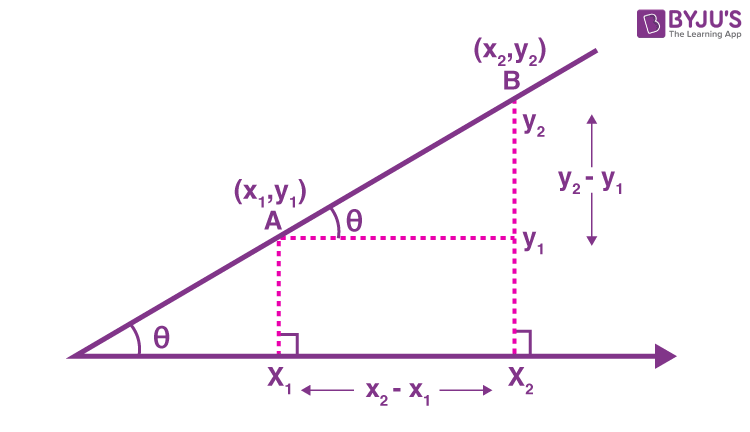### Intercept Form

The equation of the line with x-intercept as ‘a’ and y-intercept as ‘b’ can be written as;

$$\begin{array}{l}\frac{x}{a} + \frac{y}{b}=1\end{array}$$
• x – coordinate of the point of intersection of the line with the x-axis is called the x-intercept
• y-intercept will be the y-coordinate of the point of intersection of the line with the y-axis

For example,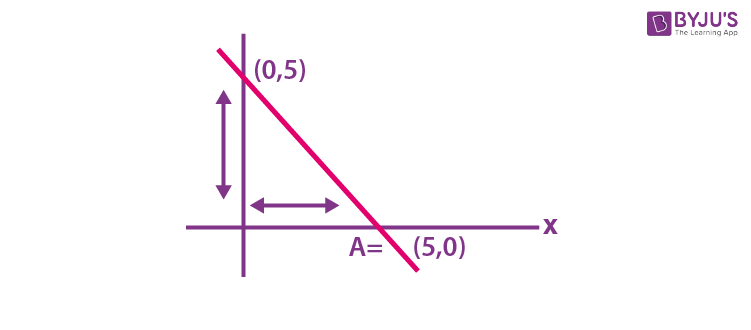Along the x-axis: x – Intercept = 5 and y – Intercept = 0

Along y-axis: y – Intercept = 5 and x – Intercept = 0

Also,

Length of x-intercept = |x1|

Length of y-intercept = |y1 |

Note: Line passes through the origin, intercept = 0

x – Intercept = 0

y – Intercept = 0

Again,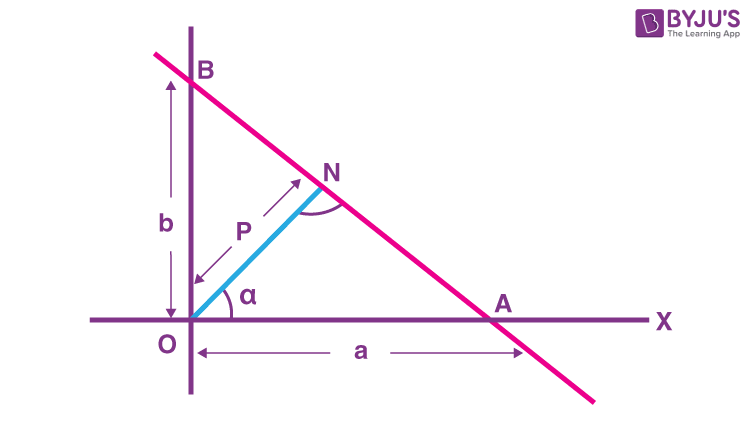ON = P

∠AON = α

Let the length of the perpendicular from origin to straight line be ‘P’ and let this perpendicular make an angle with + vex- axis ‘α’, then the equation of a line can be:

$$\begin{array}{l}x\cos \alpha +y\sin \alpha =p\end{array}$$
$$\begin{array}{l}\frac{x}{p\sec \alpha }+\frac{y}{p\cos ec\alpha }=1\end{array}$$
$$\begin{array}{l}x\cos \alpha +y\sin \alpha =P\end{array}$$

### Point form

Equation of line with slope ‘m’ and which passes through (x1, y1) can be given as

y – y= m(x – x1 )

### Slope Point form (Equation of a Line with 2 Points)

Equation of a line passing through two points (x1, y1) & (x2, y2) is given as

$$\begin{array}{l}y-{{y}_{1}} = \left( \frac{{{y}_{2}}-{{y}_{1}}}{{{x}_{2}}-{{x}_{1}}} \right)\left( x-{{x}_{1}} \right)\end{array}$$

Example: Find the equation of the line that passes through the points (-2, 4) and (1, 2).

Solution:

We know that the general equation of a line passing through two points is:

$$\begin{array}{l}y-{{y}_{1}} = \left( \frac{{{y}_{2}}-{{y}_{1}}}{{{x}_{2}}-{{x}_{1}}} \right)\left( x-{{x}_{1}} \right)\end{array}$$

Now,

(y2 – y1)/(x2 – x1) = (2 – 4)/(1 – (-2)) = -2/3

Thus, the equation of the line is:

y – 4 = (-2/3)[x – (-2)]

3(y – 4) = -2(x + 2)

3y – 12 = -2x – 4

2x + 3y – 8 = 0

Which is the required equation of the line.

## Relation between Two Lines

Let L1 and L2 be the two lines as

L1 : a1x + b1y + c1 = 0

L2  : a2x + b2y + c2 = 0

• For parallel lines

Two lines are said to be parallel if the below condition is satisfied,

$$\begin{array}{l}\frac{{{a}_{1}}}{{{a}_{2}}}=\frac{{{b}_{1}}}{{{b}_{2}}} \neq \frac{{{c}_{1}}}{{{c}_{2}}}\end{array}$$
• For intersecting lines

Two lines intersect at a point if

$$\begin{array}{l}\frac{{{a}_{1}}}{{{a}_{2}}} \neq \frac{{{b}_{1}}}{{{b}_{2}}}\end{array}$$
• For coincident lines

Two lines coincide if

$$\begin{array}{l}\frac{{{a}_{1}}}{{{a}_{2}}}=\frac{{{b}_{1}}}{{{b}_{2}}}=\frac{{{c}_{1}}}{{{c}_{2}}}\end{array}$$

### Angle between Straight Lines

$$\begin{array}{l}Let\,\,\,\,{{L}_{1}}\,\,\,\,\equiv \,\,\,y={{m}_{1}}x+{{c}_{1}}\end{array}$$
and

$$\begin{array}{l}{{L}_{2}}\,\,\,\equiv \,\,\,y={{m}_{2}}x+{{c}_{2}}\end{array}$$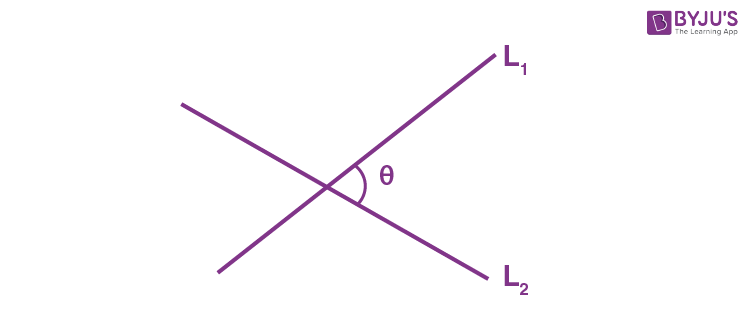$$\begin{array}{l}\text{Angle} = \theta ={{\tan }^{-1}}\left| \left( \frac{{{m}_{2}}-{{m}_{1}}}{1+{{m}_{1}}{{m}_{2}}} \right) \right|\end{array}$$

Special Cases:

$$\begin{array}{l}\Rightarrow {{m}_{2}}={{m}_{1}}\,\,\,\,\,\,\,\to \,\,\,\,\,lines\,are\,parallel\\\end{array}$$
$$\begin{array}{l}\Rightarrow \,\,{{m}_{1}}{{m}_{2}}=-1,\,\,\,\,\,\,\,\,\,\,lines\,L1\,\And L2\,are\,perpendicular\,to\,each\,other\end{array}$$

### Length of Perpendicular from a Point on a Line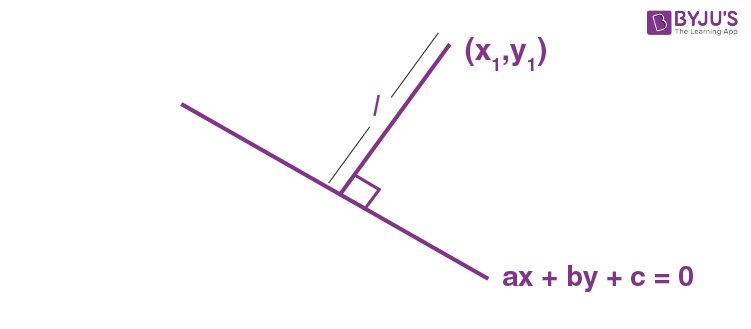The length of the perpendicular from P(x1, y1) on ax + by + c = 0 is

$$\begin{array}{l}\ell =\left| \frac{a{{x}_{1}}+b{{y}_{1}}+c}{\sqrt{{{a}^{2}}+{{b}^{2}}}} \right|\end{array}$$

B (x, y) is the foot of perpendicular is given by

$$\begin{array}{l}\frac{x-{{x}_{1}}}{a}=\frac{y-{{y}_{1}}}{b}=\frac{-(a{{x}_{1}}+b{{y}_{1}}+c)}{\left( {{a}^{2}}+{{b}^{2}} \right)}\end{array}$$

A’(h, k) is mirror image, given by

$$\begin{array}{l}\frac{h-{{x}_{1}}}{a}=\frac{k-{{y}_{1}}}{b}=\frac{-2(a{{x}_{1}}+b{{y}_{1}}+c)}{\left( {{a}^{2}}+{{b}^{2}} \right)}\end{array}$$

### Angular Bisector of Straight lines

An angle bisector has an equal perpendicular distance from the two given lines.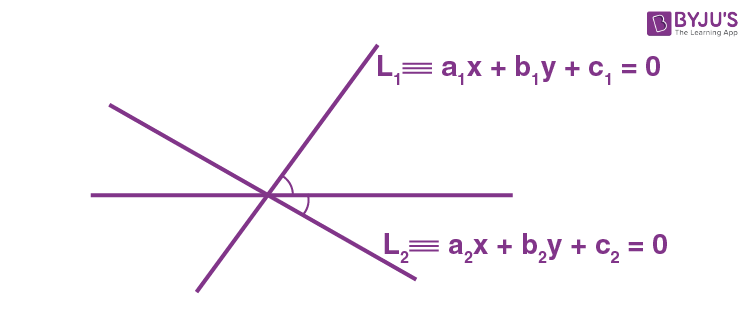The equation of line L can be given as

$$\begin{array}{l}\frac{{{a}_{1}}x+{{b}_{1}}y+{{c}_{1}}}{\sqrt{{{a}_{1}}^{2}+{{b}_{1}}^{2}}}=\pm \frac{{{a}_{2}}x+{{b}_{2}}y+{{c}_{2}}}{\sqrt{{{a}_{2}}^{2}+{{b}_{2}}^{2}}}\end{array}$$

### Family of Lines:

The general equation of the family of lines through the point of intersection of two given lines, L1 & L2, is given by L1 +λ L2 = 0

Where λ is a parameter.

### Concurrency of Three Lines

Let the lines be

$$\begin{array}{l}{{L}_{1}}\equiv {{a}_{1}}x+{{b}_{1}}y+{{c}_{1}}=0\end{array}$$
$$\begin{array}{l}{{L}_{2}}\equiv {{a}_{2}}x+{{b}_{2}}y+{{c}_{2}}=0\end{array}$$
and

$$\begin{array}{l}{{L}_{3}}\equiv {{a}_{3}}x+{{b}_{3}}y+{{c}_{3}}=0\end{array}$$

So, the condition for the concurrency of lines is

$$\begin{array}{l}\left| \begin{matrix} {{a}_{1}} & {{b}_{1}} & {{c}_{1}} \\ {{a}_{2}} & {{b}_{2}} & {{c}_{2}} \\ {{a}_{3}} & {{b}_{3}} & {{c}_{3}} \\ \end{matrix} \right|=0\end{array}$$

## Pair of Straight Lines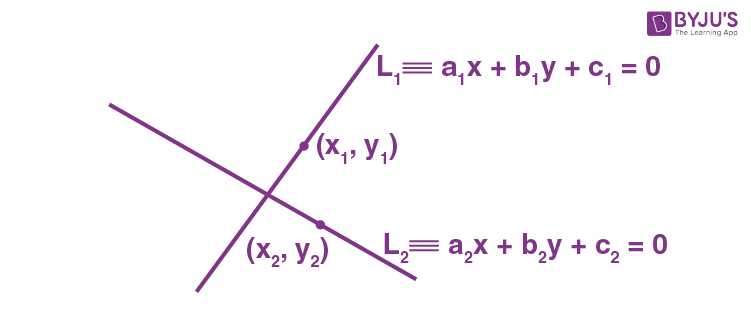Join equation of lines L1 & L2 represents P. S. L (a1x + b1y+c1) (a2x+b2y+c2) = 0

i.e. f(x,y) . g(x,y) = 0

Let’s defines a standard form of the equation:-

ax2 + by2 + 2hxy + 2gx + 2fy + c = 0 represent conics curve equation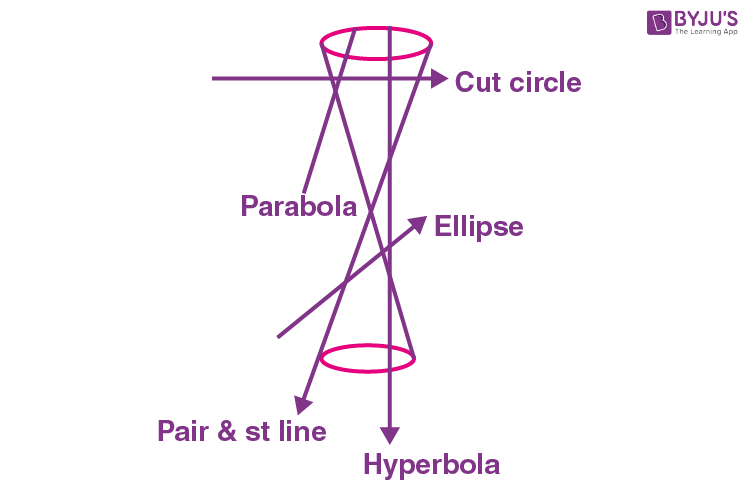Condition for curve of being P.O.S.L Δ = abc + 2fgh – af2 – bg2 – ch2 = 0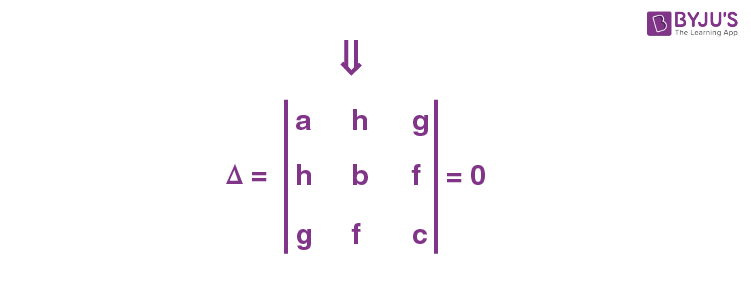If Δ ≠ 0, (i) parabola h2 = ab

(ii) hyperbola h2 < ab

(iii) circle h2 = 0, a = b

(iv) ellipse h2 > ab

Now, let’s see how did we get Δ = 0

General equation ax2 + 2gx + 2hxy + by2 + 2fy + c = 0

ax2 + (2g+2hy)x + (by2 + 2fy + c) = 0

we can consider the above equation as a quadratic equation in x, keeping y constant.

$$\begin{array}{l}x=\frac{-(2g+2hy)\pm \sqrt{{{(2g+2hy)}^{2}}-4a(b{{y}^{2}}+2fy+c)}}{2a}\end{array}$$
, so

$$\begin{array}{l}x=\frac{-(2g+2hy)\pm \sqrt{Q(y)}}{2a}\end{array}$$

Now, Q(y) has to be a perfect square, and only then, we can get two different line equations Q(y) in the perfect square for that Δ value of Q(y) should be zero.

From there D = 0

abc + 2fgh – bg2 – af2 – ch2 = 0

Or

$$\begin{array}{l}\left| \begin{matrix} a & h & g \\ h & b & f \\ g & f & c \\ \end{matrix} \right|=0\end{array}$$

Note:
1. Point of intersection

To find point of intersection of two lines (P.O.S.L), solve the P.O.S.L, factorize it in (L1).(L2) = 0 or f(x, y) . g(u,y) = 0

2. Angle between the lines

$$\begin{array}{l}\tan \theta =\left( \left| \frac{2\sqrt{{{h}^{2}}-ab}}{a+b} \right| \right)\end{array}$$

Special cases:

h2 = ab lines are either parallel or coincident

h2 < ab imaginary line

h2 > ab Two distinct lines

a + b = 0 perpendicular line

3. P.O.S.L passing through the origin, then

(y – m1x) (y – m2x) = 0

y2 – m2yx – m1xy – m1m2x2 = 0

y2 – (m1 + m2) xy – m1m2x2 = 0

ax2 + 2hxy + by2 = 0

$$\begin{array}{l}\Rightarrow {{y}^{2}}+\frac{2h}{b}xy+\frac{ab}{b}{{x}^{2}}=0\end{array}$$
$$\begin{array}{l}\Rightarrow m1 + m2 = \frac{2h}{b}\end{array}$$
$$\begin{array}{l}m_1 m_2=\frac{a}{b}\end{array}$$
$$\begin{array}{l}\tan \theta =\left| \frac{{{m}_{1}}-{{m}_{2}}}{1+{{m}_{1}}{{m}_{2}}} \right|=\left| \frac{\sqrt{{{({{m}_{1}}+{{m}_{2}})}^{2}}4{{m}_{1}}{{m}_{2}}}}{1+{{m}_{1}}{{m}_{2}}} \right|=\left| \frac{2\sqrt{{{h}^{2}}-ab}}{a+b} \right|\end{array}$$

### Straight Lines Formulas

All Formulas Related to Straight Lines
Equation of a Straight Line ax + by + c = 0
General form or Standard Form y = mx + c
Equation of a Line with 2 Points (Slope Point Form) (y – y1) = m(x – x1)

Here, m = (y2 – y1)/(x2 – x1)

Angle between Straight Lines
$$\begin{array}{l}\theta ={{\tan }^{-1}}\left| \left( \frac{{{m}_{2}}-{{m}_{1}}}{1+{{m}_{1}}{{m}_{2}}} \right) \right|\end{array}$$

## Problems on Straight Lines

Question 1:

Find the equation to the straight line which passes through the point (-5, 4) and is such that the portion of it between the axes is divided by the given point in the ratio 1 : 2.

Solution:

Let the required straight line be:

$$\begin{array}{l}\frac{x}{a}+\frac{y}{b}=1\end{array}$$

Using the given conditions,

$$\begin{array}{l}P\left( \frac{2a+1.0}{2+1},\frac{2.0+1.b}{2+1} \right)\end{array}$$
is the point which divides (a, 0) and (0, b) internally in the ratio 1 : 2.

But P is (-5, 4)

Hence, -5 = 2a/3, 4 = b/3

a = -15/2, b = 12.

Hence, the required equation is;

$$\begin{array}{l}\frac{x}{\left( -15/2 \right)}+\frac{y}{12}=1\end{array}$$

Question 2:

Find the equation of the straight line which passes through the point (1, 2) and makes an angle θ with the positive direction of the x-axis where cos θ = -1/3.

Solution:

Here cos θ = -1/3. (a negative number) so that π/2 < θ < π

$$\begin{array}{l}\Rightarrow \tan \theta =-\sqrt{8}\end{array}$$
= slope of line

We know that the equation of the straight line passing through the point (x1, y1) having slope m is

y – y1 = m(x – x1)

Therefore, the equation of the required line is

$$\begin{array}{l}y-2=-\sqrt{8}\left( x-1 \right)\end{array}$$
$$\begin{array}{l}\Rightarrow \sqrt{8}x+y-\sqrt{8}-2=0.\end{array}$$

Question 3:

Find the equation of the line joining the points (-1, 3) and (4, -2).

Solution:

Equation of the line passing through the points (x1, y1) and (x2, y2) is

$$\begin{array}{l}y-{{y}_{1}}=\frac{{{y}_{2}}-{{y}_{1}}}{{{x}_{2}}-{{x}_{1}}}\left( x-{{x}_{1}} \right)\end{array}$$

Hence, the equation of the required line will be

$$\begin{array}{l}y-3=\frac{-2 – 3}{4 + 1}\left( x+1 \right)\Rightarrow x+y-2=0\end{array}$$

Question 4:

Which line is having greatest inclination with positive direction of x-axis?

(i) Line joining points (1, 3) and (4, 7)

(ii) Line 3x – 4y + 3 = 0

Solution:

(i) Slope of line joining points A(1, 3) and B(4, 7) is

$$\begin{array}{l}\frac{7-3}{4-1}=\frac{4}{3}=\tan \alpha\end{array}$$

(ii) Slope of line is

$$\begin{array}{l}-\frac{3}{-4}=\frac{3}{4}=\tan \beta\end{array}$$

Now tan α > tan β. So line (i) has more inclination.

Question 5:

The angle of the line positive direction of the x-axis is θ. The line is rotated about some point on it in an anticlockwise direction by an angle of 45°, and its slope becomes 3. Find the angle θ.

Solution:

Originally slope of the line is tan θ = m

Now the slope of the line after rotation is 3.

The angle between the old position and the new position of the lines is 45°.

∴ we have

$$\begin{array}{l}\tan 45{}^\circ =\frac{3-m}{1+3m}\end{array}$$

1 + 3m = 3 – m

4m = 2

m = 1/2 = tan θ

θ = tan-1(1/2)

Question 6:

If line 3x – ay – 1 = 0 is parallel to the line (a + 2)x – y + 3 = 0, then find the values of a.

Solution:

Slope of line 3x – ay – 1 = 0 is 3/a.

Slope of line (a + 2)x – y + 3 = 0 is (a + 2).

Since lines are parallel then we have;

a + 2 = 3/a

or

$$\begin{array}{l}{{a}^{2}}+2a-3=0\end{array}$$

or

$$\begin{array}{l}\left( a-1 \right)\left( a+3 \right)=0\end{array}$$

a = 1 or a = -3.

Question 7:

Find the value of x for which the points (x, -1), (2, 1) and (4, 5) are collinear.

Solution:

If points A(x, -1) B(2, 1), and C(4, 5) are collinear, then

Slope of AB = Slope of BC

$$\begin{array}{l}\Rightarrow \frac{1-\left( -1 \right)}{2-x}=\frac{5-1}{4-2}\end{array}$$
$$\begin{array}{l}\Rightarrow \frac{2}{2-x}=2\Rightarrow x=1\end{array}$$

Question 8:

The slope of a line is double the slope of another line. If the tangent of the angle between them is 1/3. Find the slopes of the lines.

Solution:

Let m1 and m be the slopes of the two given lines such that m1 = 2m

We know that if θ is the angle between the lines l1 and l2 with slopes m1 and m2, then

$$\begin{array}{l}\tan \theta =\left| \frac{{{m}_{2}}-{{m}_{1}}}{1+{{m}_{1}}{{m}_{2}}} \right|\end{array}$$

It is given that the tangent of the angle between the two lines is 1/3.

$$\begin{array}{l}\therefore \frac{1}{3}=\left| \frac{m-2m}{1+\left( 2m \right).m} \right|\Rightarrow \frac{1}{3}=\left| \frac{-m}{1+2{{m}^{2}}} \right|\end{array}$$
$$\begin{array}{l}\Rightarrow 2{{\left| m \right|}^{2}}-3\left| m \right|+1=0\end{array}$$
$$\begin{array}{l}\Rightarrow \left( \left| m \right|-1 \right)\left( 2\left| m \right|-1 \right)=0\end{array}$$
$$\begin{array}{l}\Rightarrow \left| m \right|=1\,\,or\,\,\left| m \right|=1/2\end{array}$$
$$\begin{array}{l}\Rightarrow \left| m \right|\pm 1\,\,or\,\,m=\pm 1/2\\\end{array}$$
Another slope will be -2, -1, 2, 1.

Question 9:

Find the equation of the line parallel to the line 3x – 4y + 2 = 0 and passing through the point (-2, 3).

Solution:

Line parallel to the line 3x – 4y + 2 = 0 is 3x – 4y + t = 0

It passes through the point (-2, 3), so 3(-2) – 4(3) + t = 0 or t = 18.

So the equation of the line is 3x – 4y + 18 = 0.

Question 10:

Find the coordinates of the foot of the perpendicular from the point (-1, 3) to the line 3x – 4y – 16 = 0.

Solution:

Let (a, b) be the coordinates of the foot of the perpendicular from the point (-1, 3) to the line 3x – 4y – 16 = 0.Slope of the line joining (-1, 3) and (a, b)

$$\begin{array}{l}{{m}_{1}}=\frac{b-3}{a+1}\end{array}$$

Slope of the line 3x – 4y – 16 = 0 is 3/4.

Since these two lines are perpendicular, m1m2 = -1

$$\begin{array}{l}\therefore \left( \frac{b-3}{a+1} \right)\times \left( \frac{3}{4} \right)=-1\end{array}$$

⇒ 4a + 3b = 5 ….(1)

Point (a, b) lies on line 3x – 4y = 16.

⇒ 3a – 4b = 16 ….(2)

On solving equations (1) and (2), we obtain

$$\begin{array}{l}a=\frac{68}{25}\end{array}$$
and
$$\begin{array}{l}b=-\frac{49}{25}\end{array}$$

Thus, the required coordinates of the foot of the perpendicular are (68/25, -49/25).

Question 11:

Three lines x + 2y + 3 = 0, x + 2y – 7 = 0, and 2x – y – 4 = 0 form 3 sides of two squares.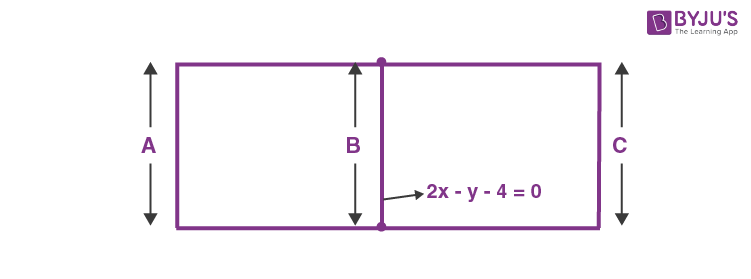Find the equations of the remaining sides of these squares.

Solution:

Distance between the two parallel lines is

$$\begin{array}{l}\frac{\left| 7+3 \right|}{\sqrt{5}}=2\sqrt{5}.\end{array}$$

The equations of the sides forming the square are of the form 2x – y + k = 0.

Since the distance between sides A and B = Distance between sides B and C

$$\begin{array}{l}\frac{\left| k-\left( -4 \right) \right|}{\sqrt{5}}=2\sqrt{5}\Rightarrow \frac{k+4}{\sqrt{5}}=\pm 2\sqrt{5}\Rightarrow k=6,-14.\end{array}$$

Hence, the fourth side of the two squares is

(i) 2x – y + 6 = 0

or

(ii) 2x – y – 14 = 0

Question 12:

For the straight lines 4x + 3y – 6 = 0 and 5x + 12y + 9 = 0, find the equation of the

(i) bisector of the obtuse angle between them

(ii) bisector of the acute angle between them

(iii) bisector of the angle which contains (1, 2)

Solution:

Equations of bisectors of the angles between the given lines are

$$\begin{array}{l}\frac{4x+3y-6}{\sqrt{{{4}^{2}}+{{3}^{2}}}}=\pm \frac{5x+12y+9}{\sqrt{{{5}^{2}}+{{12}^{2}}}}\end{array}$$

9x – 7y – 41 = 0 and 7x + 9y – 3 = 0

If θ is the angle between the line 4x + 3y – 6 = 0 and the bisector 9x – 7y – 41 = 0, then

$$\begin{array}{l}\tan \theta =\left| \frac{-\frac{4}{3}-\frac{9}{7}}{1+\left( \frac{-4}{3} \right)\frac{9}{7}} \right|=\frac{11}{3}>1.\end{array}$$

Hence

(i) The bisector of the obtuse angle is 9x – 7y – 41 = 0.

(ii) The bisector of the acute angle is 7x + 9y – 3 = 0.

(iii) For the point (1, 2)

$$\begin{array}{l}4x+3y-6=4\times 1+3\times 2-6>0,\end{array}$$
$$\begin{array}{l}5x+12y+9=5\times 1+12\times 2+9>0.\end{array}$$

Hence, equation of the bisector of the angle containing the point (1, 2) is

$$\begin{array}{l}\frac{4x+3y-6}{5}=\frac{5x+12y+9}{13}\Rightarrow 9x-7y-41=0.\end{array}$$

Question 13:

Find the value of λ if 2x2 + 7xy + 3y2 + 8x + 14y + λ = 0 will represent a pair of straight lines.

Solution:

The given equation ax2 + 2hxy + by2 + 2gx + 2fy + c = 0 represents a pair of lines if its descriminant  = 0.

i.e., if abc + 2fgh – af2 – bg2 – ch2 = 0

$$\begin{array}{l}6\lambda +2\left( 7 \right)\left( 4 \right)\left( \frac{7}{2} \right)-2{{\left( 7 \right)}^{2}}-3{{\left( 4 \right)}^{2}}-\lambda {{\left( \frac{7}{2} \right)}^{2}}=0\end{array}$$
$$\begin{array}{l}\Rightarrow 6\lambda +196-98-48-\frac{49\lambda }{4}=0\end{array}$$
$$\begin{array}{l}\Rightarrow \frac{49\lambda }{4}-6\lambda =196-146=50\end{array}$$
$$\begin{array}{l}\Rightarrow \frac{25\lambda }{4}=50\,\,\\\lambda =\frac{200}{25}=8\end{array}$$

Question 14:

If one of the lines of the pair ax2 + 2hxy + by2 = 0 bisects the angle between the positive direction of the axes, then find the relation for a, b and h.

Solution:

Bisector of the angle between the positive directions of the axes is y = x.

Since it is one of the lines of the given pair of lines ax2 + 2hxy + by2 = 0.

Let’s apply y = x.

We have

$$\begin{array}{l}{{x}^{2}}\left( a+2h+b \right)=0\end{array}$$
or
$$\begin{array}{l}a+b=-2h.\end{array}$$

Question 15:

If the angle between the two lines is represented by 2x2 + 5xy + 3y2 + 6x + 7y + 4 = 0 is tan-1(m), then find the value of m.

Solution:

The angle between the lines 2x2 + 5xy + 3y2 + 6x + 7y + 4 = 0 is given by

$$\begin{array}{l}\tan \theta =\frac{\pm 2\sqrt{\frac{25}{4}-6}}{2+3}\\ \theta ={{\tan }^{-1}}\left|( \frac{1}{5} \right)|\end{array}$$

Question 16:

The pair of lines √3 x2 – 4xy + √3 y2 = 0 are rotated about the origin by π/6 in the anticlockwise sense. Find the equation of the pair in the new position.

Solution:

The given equation of pair of straight lines can be rewritten as

$$\begin{array}{l}\left( \sqrt{3}x-y \right)\left( x-\sqrt{3}y \right)=0.\end{array}$$
Their separate equations are y = √3 x and y = (1/√3)x

or y = tan 60° x and y = tan 30° x

After rotation, the separate equations are

y = tan 90° x and y = tan 60° x

or x = 0 and y = √3 x

The combined equation in the new position is

$$\begin{array}{l}x\left( \sqrt{3}x-y \right)=0\end{array}$$
or
$$\begin{array}{l}\sqrt{3}{{x}^{2}}-xy=0\end{array}$$

## Video Lessons

### Straight Line – Short Notes and Important Formulas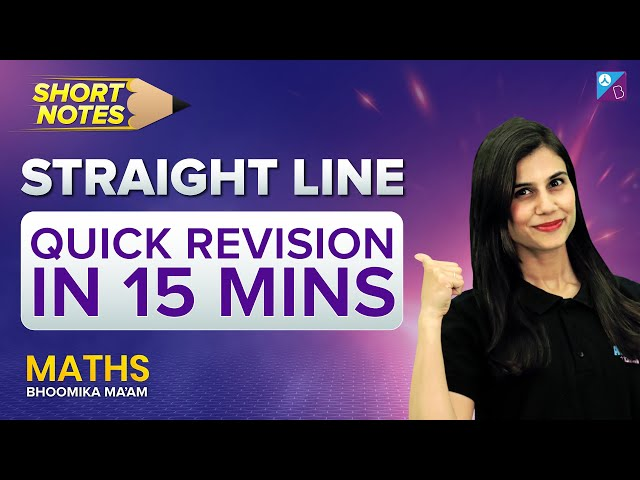### Straight Lines & Circles JEE Advanced Questions### Straight Lines Video Lesson### Straight Lines Problems and Solutions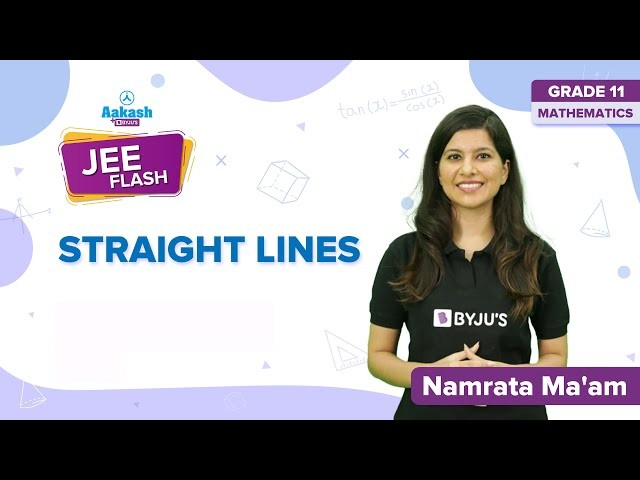### Straight Lines – Important JEE Main Questions### Straight Lines and Circles – Top 12 Important QuestionsQ1

### Give the general equation of a straight line.

The general equation of a straight line is ax + by + c = 0. (x, and y are variables and a, b, c are constants.)

Q2

### What do you mean by the slope of a straight line?

The angle formed by a line with a positive x-axis is the slope of a line. It is denoted by tan θ.

Q3

### What is the equation of the straight line passing through (x1, y1) with slope m?

The equation of the straight line passing through (x1, y1) with slope m is given by y – y1 = m(x – x1 ).

Test your Knowledge on Straight lines